Latest Banking jobs   »

# Quantitative Aptitude Quiz For Bank of Baroda AO 2023 -25th April

Q1. Veer invested an amount on simple interest, and it becomes two times of itself in 10 years. If Veer invested Rs. 1600 at the same rate of interest on CI, then find amount will get by Veer after three years (in Rs.)?
(a) 2100.6
(b) 2184.6
(c) 2196.6
(d) 2129.6
(e) 2164.6
Q2. A man invests a sum of 3600 in two part one at rate of 5% and other at rate of 7% and get total interest 204 as SI at the end of year. Find what part was invested at 7% rate.
(a) 1200
(b) 2400
(c) 1800
(d) 3000
(e) 600

Q3. A man invests 2500 Rs at 20% Compounded annually. Find the difference between interest earned in second year and third year.
(a) 100
(b) 220
(c) 20
(d) 120
(e) 60
Q4. A sum becomes Rs. 4840 in two years and Rs. 5856.4 in four years when compounded annually. What would be the amount if same sum invested at 15% S.I. for 3 years?
(a) 5600
(b) 5000
(c) 6400
(d) 6200
(e) 5800
Q5. Difference of the interest received when a sum is invested at 15% p.a. at SI for two years and the interest received when that sum is invested at 20% p.a. for one year compounded half yearly is Rs 432, find the sum?
(a) Rs. 5400
(b) Rs. 5000
(c) Rs. 4500
(d) Rs. 4000
(e) Rs. 4800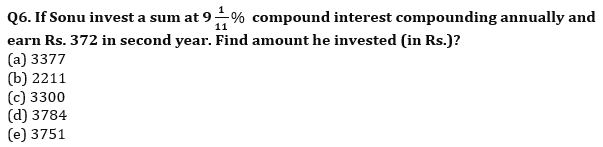Q7. On Rs 3500 invested at a simple interest rate 7 per cent per annum, Rs 500 is obtained as interest in certain years, In order to earn Rs 800 as interest on Rs 4900 in the same number of years, what should be the rate of simple interest?
(a) 9%
(b) 10%
(c) 12%
(d) 8%
(e) None of these
Q8. A mixture of milk and water is in ratio 5 : 3. If 40 liters of mixture is taken out and 4 liters of pure water is added to the mixture, then percentage of water in the mixture becomes 40%. Find the initial quantity of mixture.
(a) 148 liters
(b) 144 liters
(c) 150 liters
(d) 136 liters
(e) 140 liters
Q9. A tank contains 384 liters mixture of milk & water in the ratio of 15 : 17. If X liters of mixture taken out and (X – 14) liters of milk & (X – 34) liters of water added in remaining mixture so ratio of milk & water becomes equal. Find quantity of milk in original mixture is what percent of total final mixture?
(a) 40%
(b) 35%
(c) 45%
(d) 50%
(e) 25%
Q10. Anurag & Ravi entered into a business with capital of Rs. 2000 & Rs. 1600 respectively. At the end of 8th month Anurag added Rs. 400 more and he left the business after 10 months, after one year’s Ravi invested his profit in a scheme, which offers CI at 20% p.a. If after two years Ravi gets total amount of Rs. 6912, then find total profit of Anurag?
(a) 5200 Rs.
(b) 2550 Rs.
(c) 10200 Rs.
(d) 7650 Rs.
(e) 2700 Rs.
Q11. Ratio of investment of A to that of B is 13:25 and after 4 months, A increases his investment by 2/13 th of the initial amount, B withdraws 50% of the amount and C makes an investment. At the end of the year profit share of C is twice of the difference between profit share of A and B. Find initial investment of C is what percent of that of B?
(a) 24.5%
(b) 21%
(c) 42%
(d) 35%
(e) 28%
Q12.Veer and Anurag invested Rs. 1200 & Rs. 1600 respectively and after 15 months Veer withdrew 2/23 th of total profit, while Anurag added 4/23 th of total profit. If at the end of two years share of profit ratio of Veer & Anurag is 9 : 14, then find the difference between profit share of Veer & Anurag?
(a) Rs. 400
(b) Rs. 450
(c) Rs. 500
(d) Rs. 550
(e) Rs. 600
Q13. In a juice, guava is 85% and rest is sugar. In another juice, pomegranate is 90% while rest is sugar. Both the juices are mixed in the ratio of 3 : 2. Find concentration of sugar in final mixture. (in %)
(a) 10
(b) 11
(c) 12
(d) 13
(e) 14
Q14. Two varieties of wheat is mixed in the ratio of 2:3 by weight. The price of the mixture is Rs.12/kg and the price of the variety having lower weight is Rs 10/kg. Find the price per kg of the other variety.(in Rs)
(a) 35/3
(b)50/3
(c) 38/3
(d)47/3
(e)40/3
Q15. A container is full of milk, 1/4 th of the milk is taken out and is replaced with water, and this process is repeated 3 times and 297ℓ of milk is finally left in the container. find the capacity of container? (in ℓ)
(a) 528
(b) 644
(c) 740
(d) 704
(e) 750

Solutions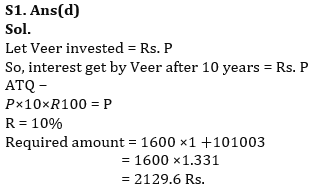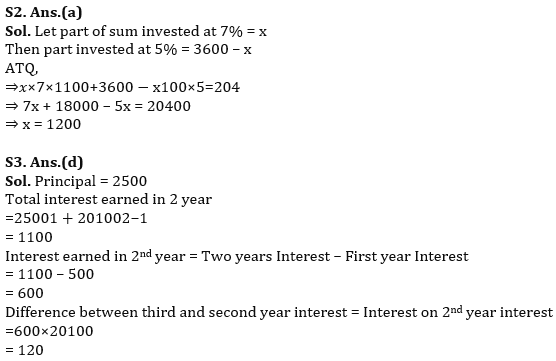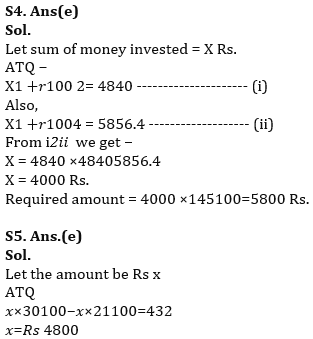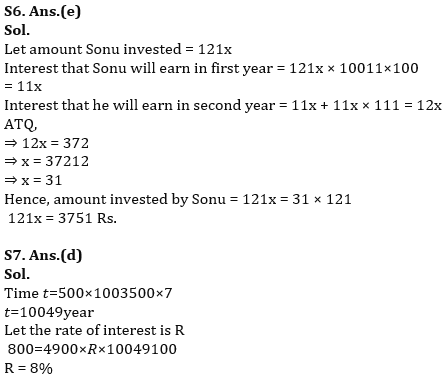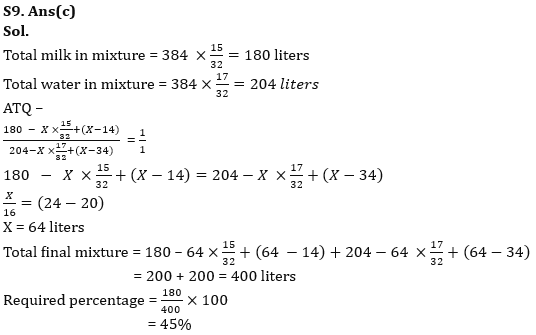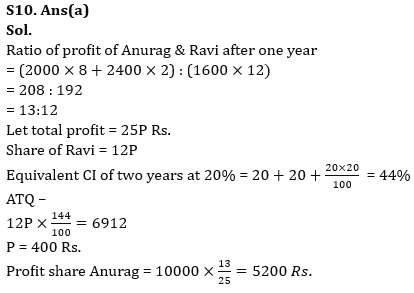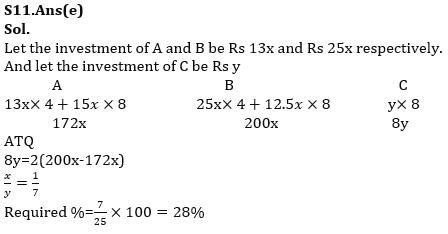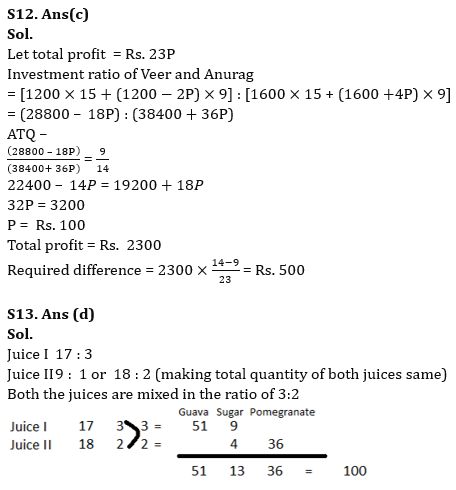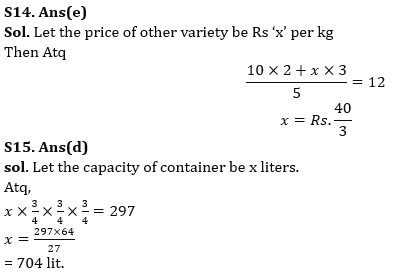## FAQs

### What is the selection process of Bank of Baroda AO 2023?

The selection process of Bank of Baroda AO consists of Online exam and Interview

#### Congratulations!Union Budget 2023-24: Free PDF